Question

# Turnbull Co. has a target capital structure of 45% debt, 4% preferred stock, and 51% common equity. It has a before-tax...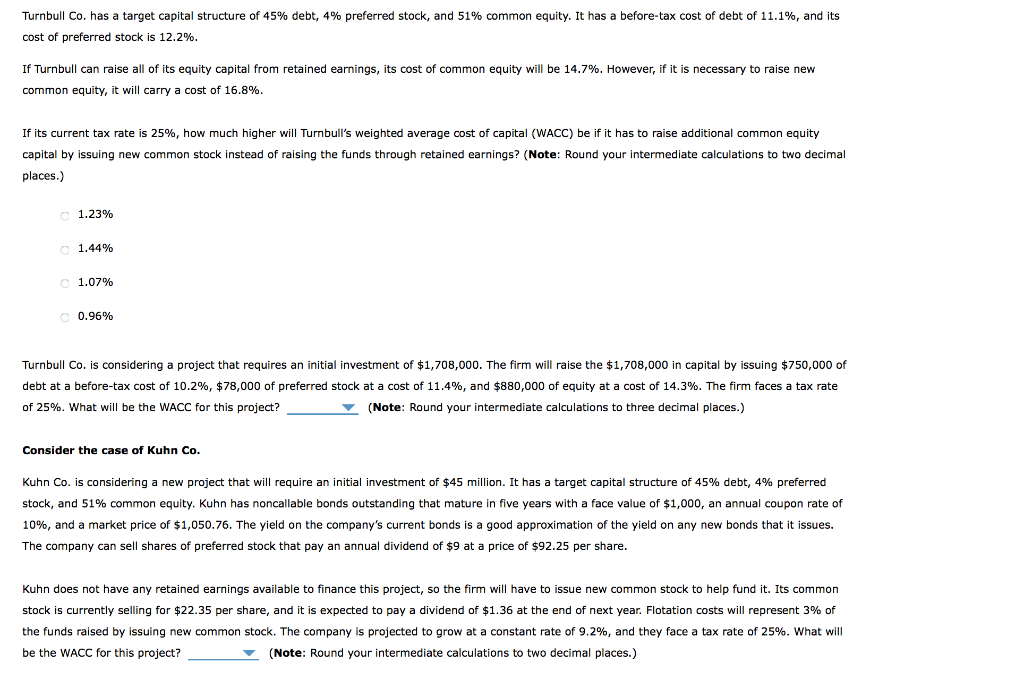Turnbull Co. has a target capital structure of 45% debt, 4% preferred stock, and 51% common equity. It has a before-tax cost of debt of 11.1%, and its cost of preferred stock is 12.2% If Turnbull can raise all of its equity capital from retained earnings, its cost of common equity will be 14.7%. However, if it is necessary to raise new common equity, it will carry a cost of 16.8%. If its current tax rate is 25%, how much higher will Turnbull's weighted average cost of capital (WACC) be if it has to raise additional common equity capital by issuing new common stock instead of raising the funds through retained earnings? (Note: Round your intermediate calculations to two decimal places.) 1.23% 1.44% 1.07% 0.96% Turnbull Co. is considering a project that requires an initial investment of \$1,708,000. The firm will raise the \$1,708,000 in capital by issuing \$750,000 of debt at a before-tax cost of 10.2%, \$78,000 of preferred stock at a cost of 11.4%, and \$880,000 of equity at a cost of 14.3%. The firm faces a tax rate of 25%. What will be the WACC for this project? (Note: Round your intermediate calculations to three decimal places.) Consider the case of Kuhn Co. Kuhn Co. is considering a new project that will require an initial investment of \$45 million. It has a target capital structure of 45% debt, 4% preferred stock, and 51% common equity. Kuhn has noncallable bonds outstanding that mature in five years with a face value of \$1,000, an annual coupon rate of 10%, and a market price of \$1,050.76. The yield on the company's current bonds is a good approximation of the yield on any new bonds that it issues. The company can sell shares of preferred stock that pay an annual dividend of \$9 at a price of \$92.25 per share. Kuhn does not have any retained earnings available to finance this project, so the firm will have to issue new common stock to help fund it. Its common stock is currently selling for \$22.35 per share, and it is expected to pay a dividend of \$1.36 at the end of next year. Flotation costs will represent 3% of the funds raised by issuing new common stock. The company is projected to grow at a constant rate of 9.2%, and they face a tax rate of 25%. What will be the WACC for this project? (Note: Round your intermediate calculations to two decimal places.)

Question 1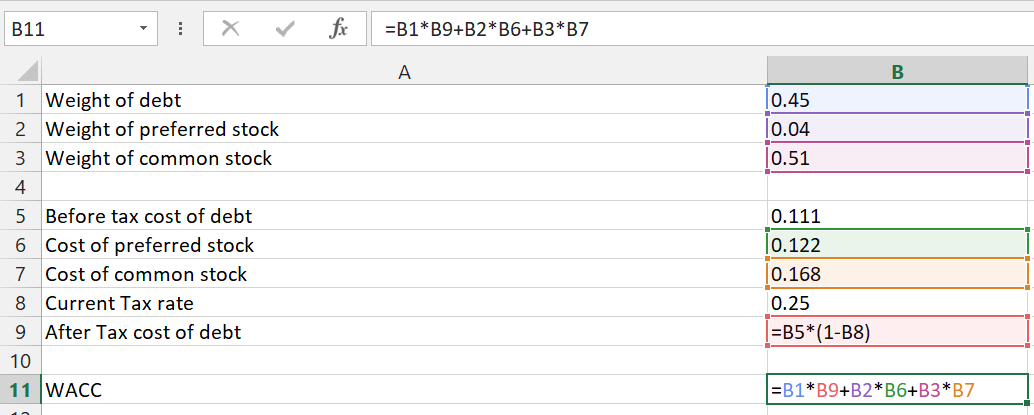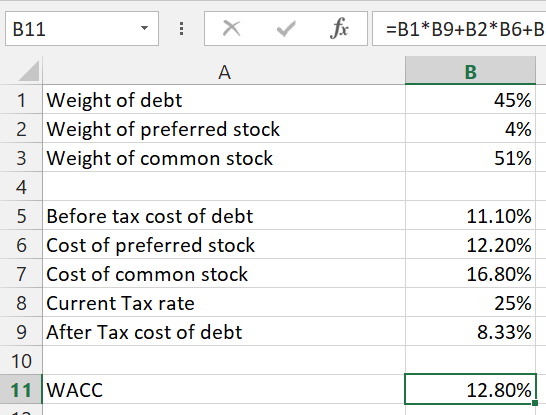NOte: As per HOMEWORKLIB POLICY only one separate question per post is answered. Please request the remaining questions in a separate post.

#### Earn Coins

Coins can be redeemed for fabulous gifts.

Similar Homework Help Questions
• ### Turnbull Co. has a target capital structure of 58% debt, 6% preferred stock, and 36% common equity. It has a before-tax...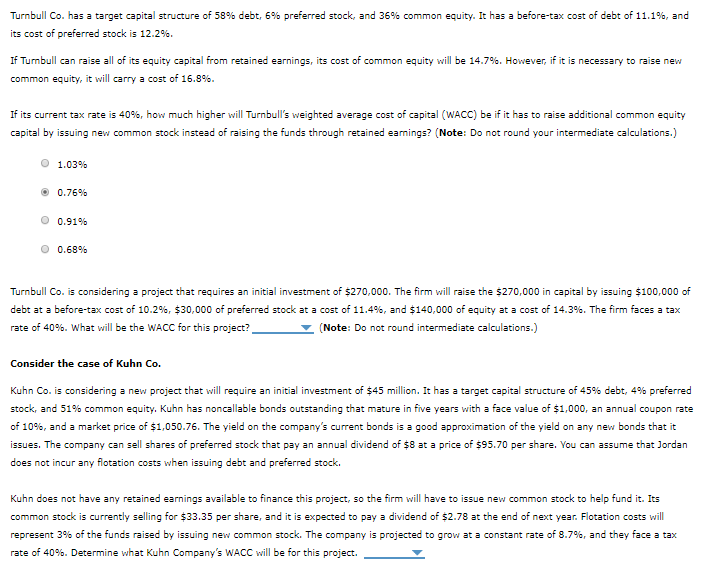Turnbull Co. has a target capital structure of 58% debt, 6% preferred stock, and 36% common equity. It has a before-tax cost of debt of 11.1%, and its cost of preferred stock is 12.2%. If Turnbull can raise all of its equity capital from retained earnings, its cost of common equity will be 14.7%. However, if it is necessary to raise new common equity, it will carry a cost of 16.8%. If its current tax rate is 40%, how much...

• ### Turnbull Co. has a target capital structure of 45% debt, 4% preferred stock, and 51% common...

Turnbull Co. has a target capital structure of 45% debt, 4% preferred stock, and 51% common equity. It has a before-tax cost of debt of 8.2%, and its cost of preferred stock is 9.3%. If Turnbull can raise all of its equity capital from retained earnings, its cost of common equity will be 12.4%. However, if it is necessary to raise new common equity, it will carry a cost of 14.2%. If its current tax rate is 40%, how much...

• ### please help Turnbull Co. has a target capital structure of 58% debt, 6% preferred stock, and...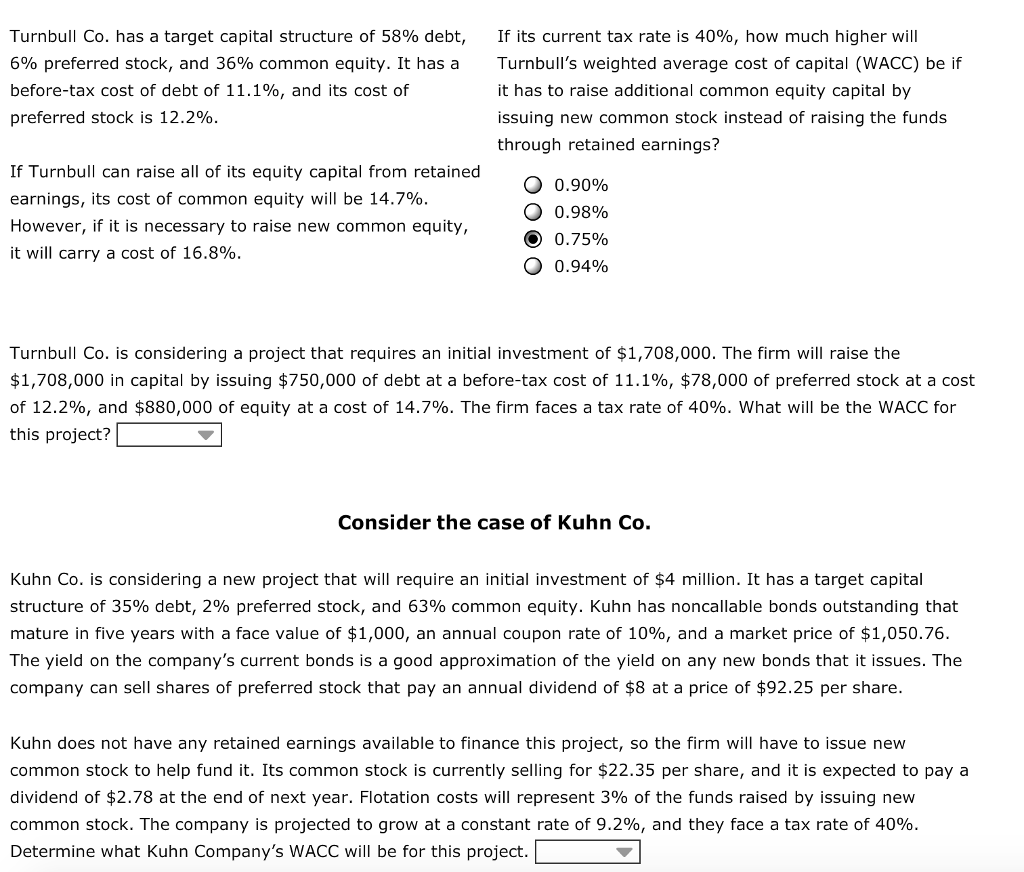please help Turnbull Co. has a target capital structure of 58% debt, 6% preferred stock, and 36% common equity. It has a before-tax cost of debt of 11.1%, and its cost of preferred stock is 12.2% if its current tax rate is 40%, how much higher will Turnbull's weighted average cost of capital (WACC) be if it has to raise additional common equity capital by issuing new common stock instead of raising the funds through retained earnings? If Turnbull can...

• ### Turnbull Co. has a target capital structure of 58% debt, 6% preferred stock, and 36% common...

Turnbull Co. has a target capital structure of 58% debt, 6% preferred stock, and 36% common equity. It has a before-tax cost of debt of 11.1%, and its cost of preferred stock is 12.2%. If Turnbull can raise all of its equity capital from retained earnings, its cost of common equity will be 14.7%. However, if it is necessary to raise new common equity, it will carry a cost of 16.8%. A) If its current tax rate is 40%, how...

• ### Turnbull Co. has a target capital structure of 58% debt, 6% preferred stock, and 36% common...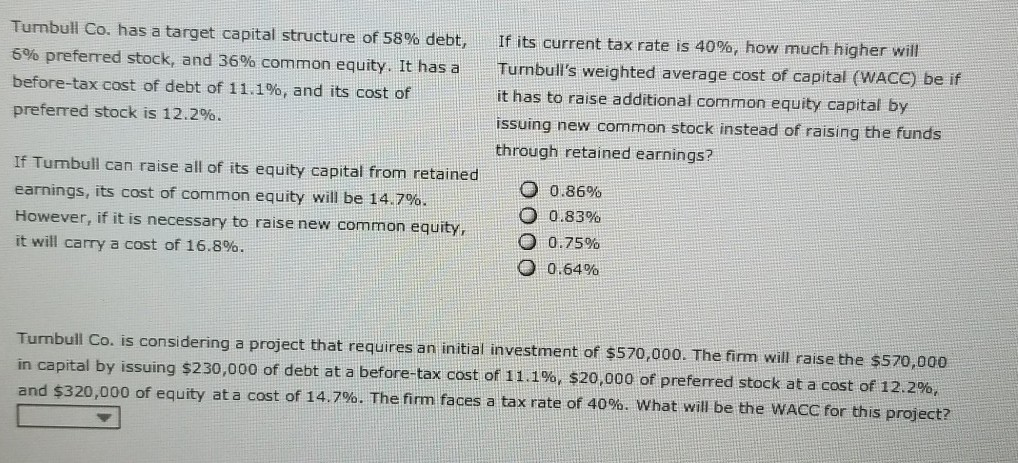Turnbull Co. has a target capital structure of 58% debt, 6% preferred stock, and 36% common equity. It has a before-tax cost of debt of 11.1%, and its cost of preferred stock is 12.2%. If its current tax rate is 40%, how much higher will Turnbull's weighted average cost of capital (WACC) be if it has to raise additional common equity capital by issuing new common stock instead of raising the funds through retained earnings? If Tumbull can raise all...

• ### Consider the case of Turnbull Co. Turnbull Co. has a target capital structure of 58% debt,...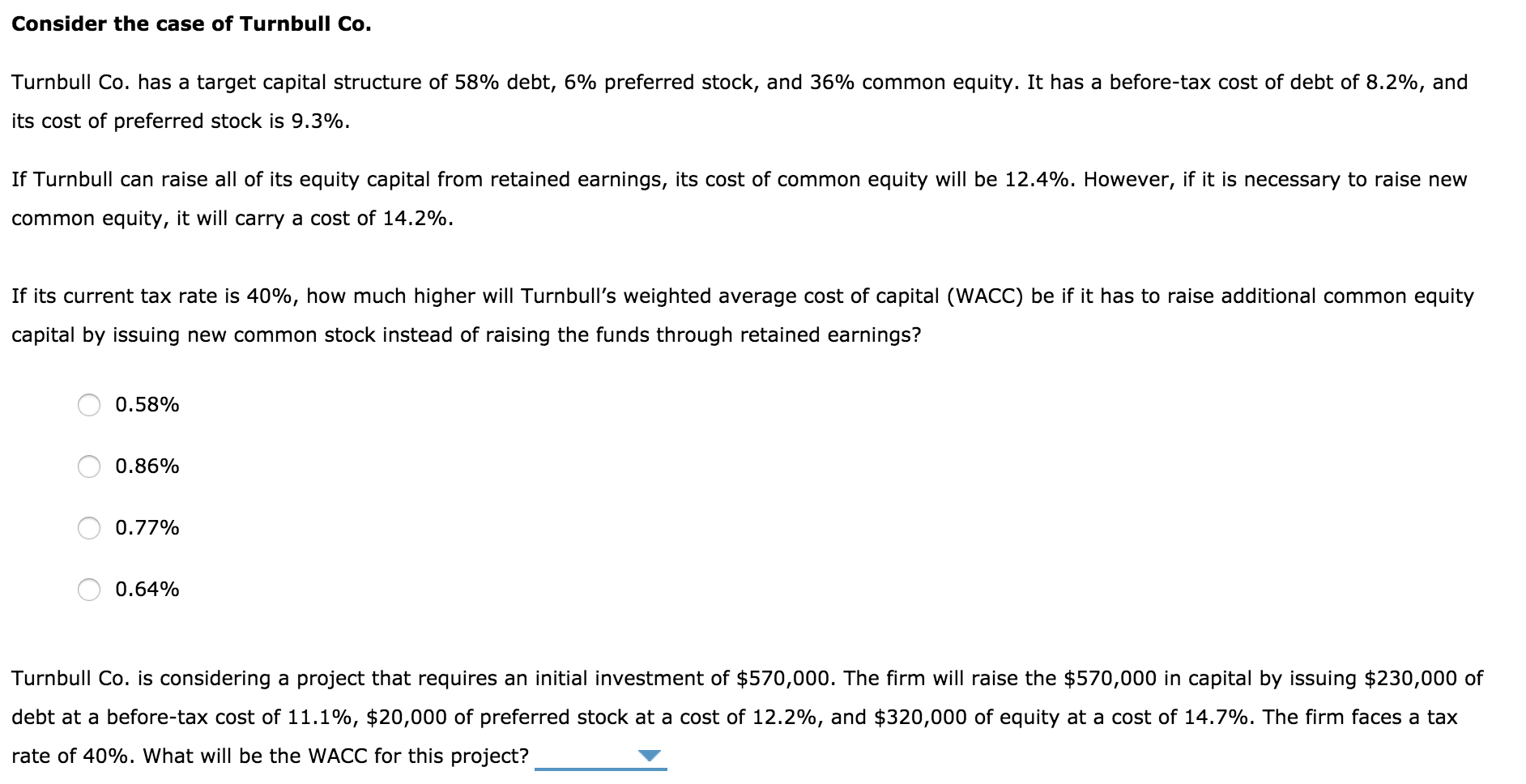Consider the case of Turnbull Co. Turnbull Co. has a target capital structure of 58% debt, 6% preferred stock, and 36% common equity. It has a before-tax cost of debt of 8.2%, and its cost of preferred stock is 9.3%. If Turnbull can raise all of its equity capital from retained earnings, its cost of common equity will be 12.4%. However, if it is necessary to raise new common equity, it will carry a cost of 14.2%. If its current...

• ### Turnbull Co. has a target capital structure of 58% debt, If its current tax rate is...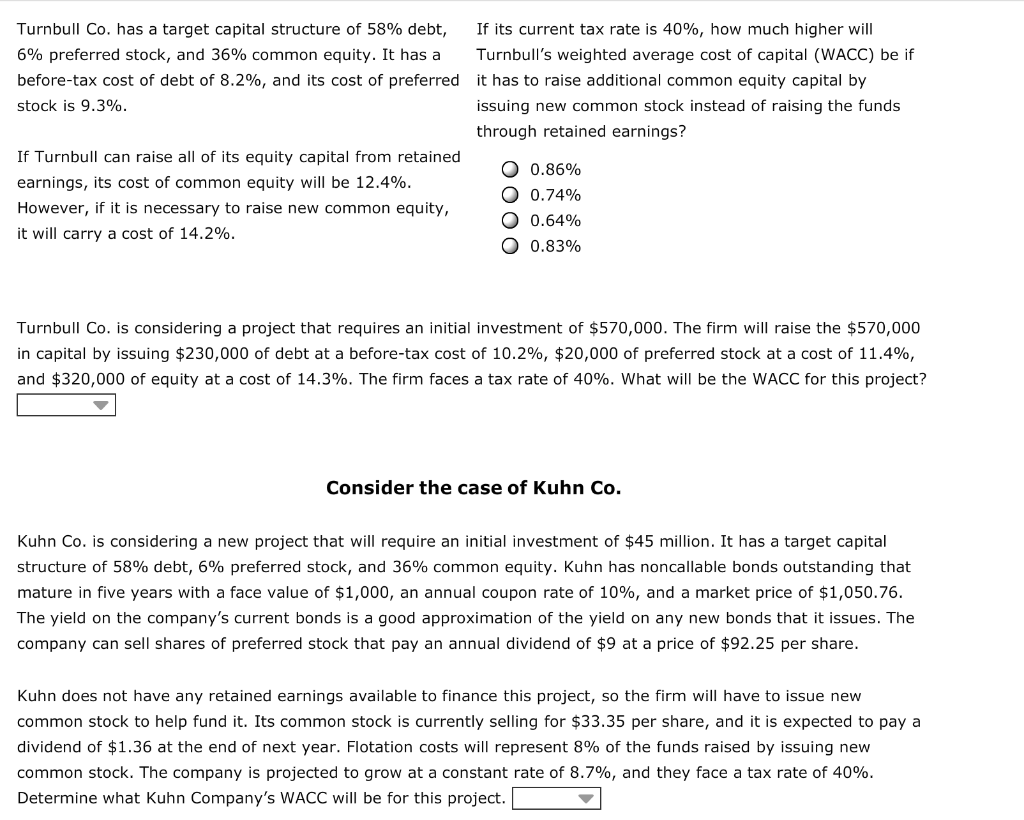Turnbull Co. has a target capital structure of 58% debt, If its current tax rate is 40%, how much higher will 6% preferred stock, and 36% common equity. It has a Turnbull's weighted average cost of capital (WACC) be if before-tax cost of debt of 8.2%, and its cost of preferred it has to raise additional common equity capital by stock is 9.3%. issuing new common stock instead of raising the funds through retained earnings? If Turnbull can raise all...

• ### Consider the case of Turnbull Co. Turnbull Co. has a target capital structure of 58% debt,...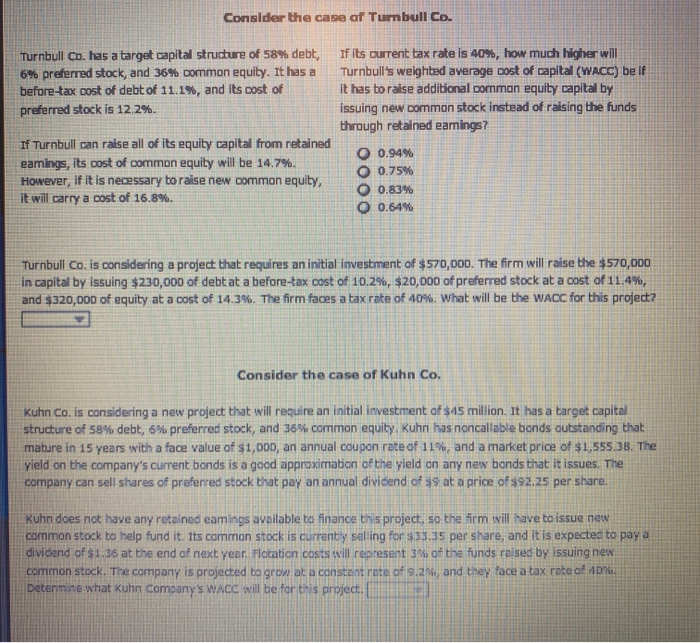Consider the case of Turnbull Co. Turnbull Co. has a target capital structure of 58% debt, 6% preferred stock, and 36% common equity. It has a before-tax cost of debt of 11.1%, and its cost of preferred stock is 12.2%. If its current tax rate is 40%, how much higher will Turnbull's weighted average cost of capital (WACC) be if it has to raise additional common equity capital by issuing new common stock instead of raising the funds through retained...

• ### Options for question 3. 12.82,13.40,12.23,11.65 Consider the case of Turnbull Co. Turnbull Co. has a target...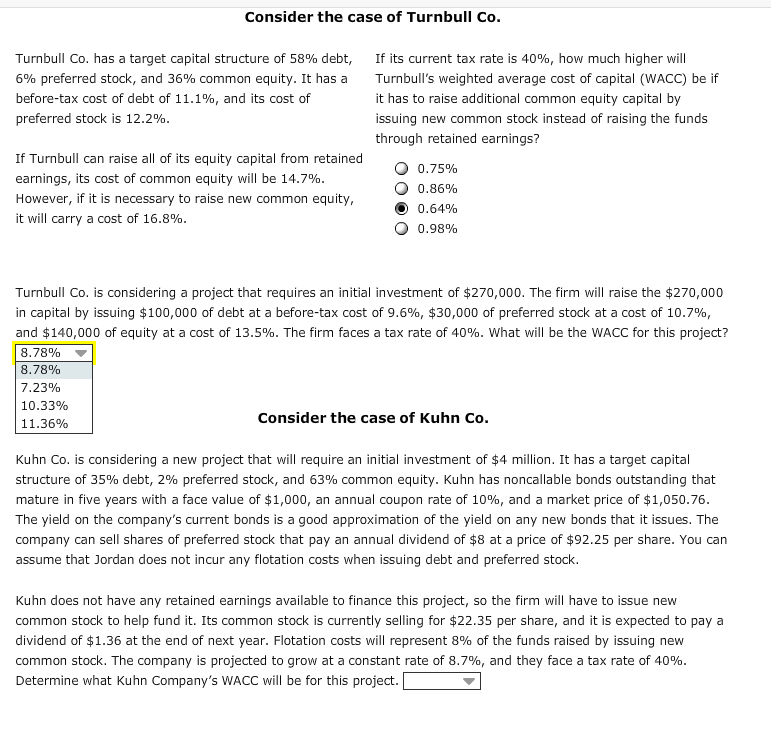Options for question 3. 12.82,13.40,12.23,11.65 Consider the case of Turnbull Co. Turnbull Co. has a target capital structure of 58% debt, 6% preferred stock, and 36% common equity. It has a before-tax cost of debt of 11.1%, and its cost of preferred stock is 12.2%. If its current tax rate is 40%, how much higher will Turnbull's weighted average cost of capital (WACC) be if it has to raise additional common equity capital by issuing new common stock instead of...